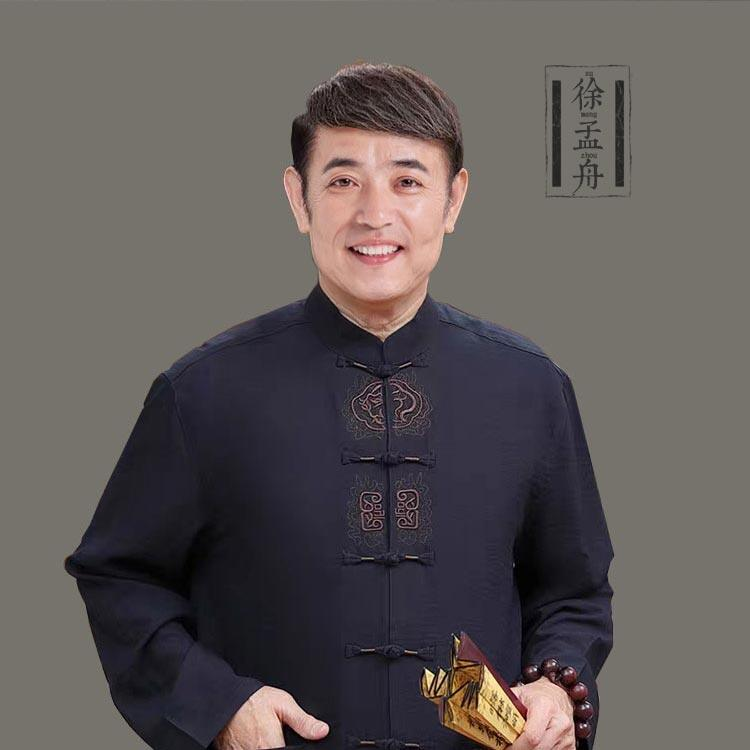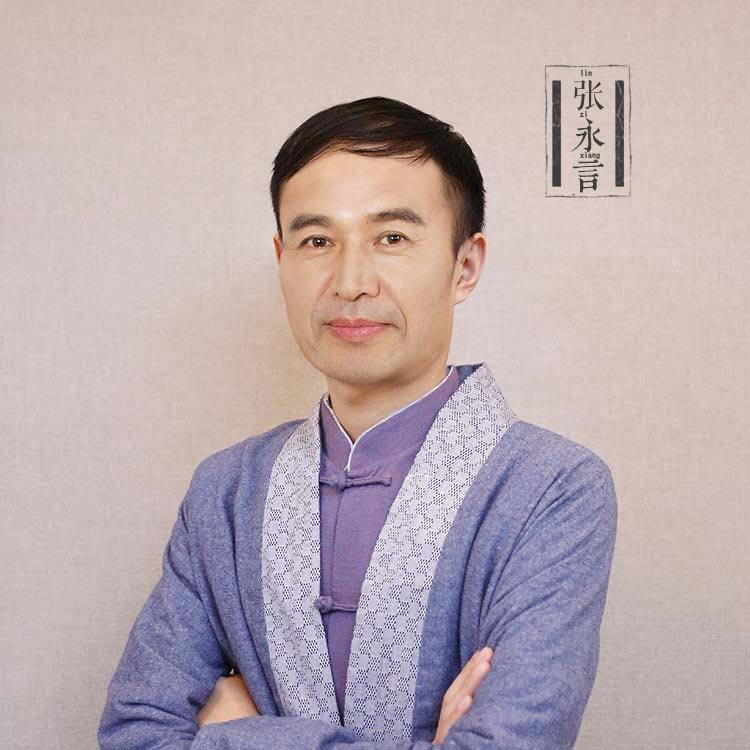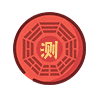# 于姓男孩名字大全_姓于的男孩名字-起名网## 于姓男孩名字大全

• 于雨泽
• 于浩然
• 于海洋
• 于海阳
• 于昆鹏
• 于鹏飞
• 于为波
• 于山珊
• 于娜威
• 于洪正
• 于佳弘
• 于雨嫄
• 于佳卉
• 于弘蕊
• 于弘月
• 于庆欣
• 于瀛童
• 于宇航
• 于曦涵
• 于静波
• 于梦涵
• 于涵宇
• 于金洪
• 于涵雨
• 于洪彰
• 于涵珊
• 于宏彰
• 于楚晗
• 于帆
• 于凡
• 于简夕
• 于卦狱
• 于成龙
• 于景天
• 于浩博
• 于景添
• 于润泽
• 于未
• 于梦瑶
• 于楚涵
• 于军兰
• 于嘉璇
• 于曦淼
• 于锦淏
• 于焱
• 于洱康
• 于力霈
• 于宝睿
• 于晨一
• 于晨曦
• 于漾煕
• 于哲瀚
• 于涵崡
• 于泽辰
• 于泽晨
• 于泽宸
• 于晨铭
• 于灏淼
• 于依凡
• 于傲雪
• 于芸
• 于雨璇
• 于鹏光
• 于炜人
• 于宏伟
• 于露
• 于昊洋
• 于逸凡
• 于博雅
• 于玥懿
• 于国俊
• 于博俊
• 于其妙
• 于子豪
• 于浩宸
• 于响训
• 于晴蓝
• 于晴兰
• 于东洋
• 于宸轩
• 于晨雨
• 于晨心
• 于果
• 于雪芸
• 于宸旭
• 于雅雯
• 于涵予
• 于金康
• 于梦昕
• 于诗艺
• 于泳浩
• 于鑫雨
• 于鑫予
• 于浩鑫
• 于宸鑫
• 于金弘
• 于鑫康
• 于宸泽
• 于宸浩
• 于宸宇
• 于宸雨
• 于博涵
• 于博瀚
• 于碑了
• 于子萱
• 于星泽
• 于鑫焱
• 于浩泽
• 于焱吉
• 于丽
• 于会香
• 于入笞
• 于米多
• 于鉴岩
• 于传昭
• 于海东
• 于倩
• 于森
• 于赢
• 于少泽
• 于千桐
• 于是
• 于泽熙
• 于嘉懿
• 于松宁
• 于佳熙
• 于佳希
• 于美辰
• 于美晨
• 于晓巍
• 于广梅
• 于子航
• 于莹
• 于阳
• 于伟逹
• 于军生
• 于民生
• 于浩
• 于敏
• 于越
• 于国松
• 于金永
• 于金方
• 于召亮
• 于俊熙
• 于峻熙
• 于浩轩
• 于佳琪
• 于欣妍
• 于丹丹
• 于子淇
• 于文哲
• 于冰清
• 于玉洁
• 于萍
• 于逸轩
• 于子轩
• 于汶杰
• 于子祥
• 于珈
• 于皓喆
• 于博超
• 于俏颜
• 于郑洋
• 于海涛
• 于文静
• 于子宸
• 于婧欣
• 于舒蓉
• 于静璇
• 于靖欣
• 于舒婧
• 于婧舒
• 于静轩
• 于筱彤
• 于晓倩
• 于晓彤
• 于舒蓓
• 于舒靖
• 于靖舒
• 于爱园
• 于全文
• 于昊天
• 于洋
• 于涛
• 于金灵
• 于金枝
• 于宝枝
• 于佳琦
• 于海杰
• 于泽涵
• 于彦博
• 于彦杰
• 于浩铭
• 于浩明
• 于文卓
• 于慕涵
• 于文博
• 于文熙
• 于文泽
• 于竟飞
• 于牧飞
• 于海滨
• 于木易
• 于德铭
• 于振洋
• 于正洋
• 于德拥
• 于德浩
• 于顺林
• 于灏暄
• 于灏涛
• 于灏苒
• 于灏悦
• 于佳城
• 于佳诚
• 于佳成
• 于嘉成
• 于嘉堉
• 于嘉骋
• 于嘉诚
• 于家成
• 于家城
• 于钿宝
• 于添宝
• 于俊哲
• 于俊泽
• 于恒溢
• 于恒毅
• 于雷
• 于子昂
• 于旭
• 于晨
• 于丹妮
• 于晨轩
• 于皓轩
• 于晨智
• 于飞轩
• 于晨锋
• 于晨升
• 于晨容
• 于晨荣
• 于晨翔
• 于晨麟
• 于晨轩
• 于晨明
• 于晨阳
• 于炎
• 于萧菡
• 于湘业
• 于谦
• 于腾腾
• 于一诺
• 于乐乐
• 于家乐
• 于浪
• 于方百
• 于长卿
• 于小伟
• 于凤舞
• 于腾空
• 于睡觉
• 于腾飞
• 于龙飞
• 于龍飞
• 于星腾
• 于腾逸
• 于腾骏
• 于腾轩
• 于天赐
• 于梦梦
• 于升伟
• 于小闯
• 于艺文
• 于天龙
• 于添龙
• 于腾波
• 于茹梦
• 于小鱼
• 于龙霄
• 于龙啸
• 于姣
• 于海龙
• 于焕迁
• 于文萍
• 于艺昕
• 于士恩
• 于懿涵
• 于舒然
• 于凼涵
• 于子源
• 于霄涵
• 于子雯
• 于子涵
• 于小雅
• 于晔
• 于自孝
• 于英辉
• 于源祺
• 于君龙
• 于昊炜
• 于潇然
• 于杨熙
• 于洋熙
• 于漾熙
• 于漾希
• 于杨希
• 于森淼
• 于嵩涛
• 于木子
• 于熙来
• 于丁
• 于瀚博
• 于冉
• 于熙
• 于豫
• 于锦熙
• 于熙旺
• 于乾熙
• 于银熙
• 于钱熙
• 于熙哲
• 于土哲
• 于哲
• 于祥
• 于鑫涛
• 于嘉鑫
• 于嘉歆
• 于泽
• 于天骄
• 于怡熙
• 于慕晴
• 于沐熙
• 于一航
• 于晓冉
• 于艺涵
• 于佳涊
• 于瑷菡
• 于留洋
• 于元博
• 于二华
• 于双华
• 于痕
• 于优酷
• 于清宁
• 于珺晗
• 于亮
• 于文凯
• 于文畅
• 于添夷
• 于添太
• 于子桐
• 于铭辛
• 于辛淇
• 于斌
• 于美芬
• 于了泵
• 于邝壤
• 于笼喾
• 于小桐
• 于爱敏
• 于敬冉
• 于蓓儿
• 于夕儿
• 于若夕
• 于思涵
• 于元颢
• 于元皓
• 于元浩
• 于升阳
• 于元坤
• 于梓焱
• 于浩鸣
• 于浠尧
• 于瀚尧
• 于铭喆
• 于瑷连
• 于丁一
• 于塬颢
• 于塬博
• 于垣博
• 于源博
• 于源地
• 于芳
• 于源泓
• 于海益
• 于海伊
• 于海宜
• 于海亦
• 于海
• 于海奕
• 于海毅
• 于海逸
• 于海轶
• 于海怡
• 于海一
• 于海仪
• 于海艺
• 于海依
• 于海亿
• 于海翼
• 于胡杨
• 于元昊
• 于富
• 于源灏
• 于溧灿
• 于大川
• 于浩予
• 于涣辰
• 于浩宇
• 于沛晨
• 于梓浩
• 于沛辰
• 于浩涵
• 于昊涵
• 于浩洋
• 于盛泽
• 于浩瀚
• 于宏萌
• 于宏文
• 于宏惠
• 于宏彤
• 于宏莹
• 于宏欣
• 于宏慈
• 于宏静
• 于宏蕊
• 于宏冉
• 于宏娜
• 于宏馨
• 于宏新
• 于艺轩
• 于震华
• 于嘉禾
• 于子珊
• 于中华
• 于小越
• 于昕艳
• 于庆胜
• 于杨
• 于琭睡
• 于夕娟
• 于靚晨
• 于靓晨
• 于亮晨
• 于敏芝
• 于义泽
• 于兆亮
• 于跃洋
• 于昕洋
• 于昕泽
• 于昕治
• 于昕至
• 于子妍
• 于吉学
• 于皓阳
• 于志刚
• 于淑青
• 于博翔
• 于莺
• 于莹莹
• 于蕴莹
• 于韵莹
• 于芸莹
• 于利莹
• 于丽莹
• 于丽莺
• 于感又
• 于红
• 于俊杰
• 于庆沣
• 于贺澎
• 于荣光
• 于海霞
• 于骏臣
• 于靓
• 于子程
• 于子成
• 于清溪
• 于艺可
• 于一彤
• 于松楠
• 于驰逸
• 于辰骏
• 于曦辰
• 于子辰
• 于天佑
• 于佳轩
• 于佳町
• 于佳欣
• 于佳鑫
• 于朋宗
• 于朋泽
• 于朋潇
• 于朋曦
• 于朋晟
• 于朋郝
• 于朋劭
• 于朋邵
• 于朋睿
• 于朋芮
• 于朋铷
• 于朋岭
• 于朋翎
• 于朋亮
• 于朋葵
• 于朋凯
• 于朋兢
• 于朋珲
• 于朋蔚
• 于朋蔷
• 于朋磊
• 于朋辉
• 于朋晖
• 于朋皓
• 于朋浩
• 于朋海
• 于朋茱
• 于朋诰
• 于朋罡
• 于朋德
• 于朋程
• 于朋菖
• 于朋昌
• 于朋博
• 于朋垒
• 于朋蕾
• 于朋健
• 于朋涛
• 于朋建
• 于鹏涛
• 于紫嫣
• 于紫萱
• 于鸾鳙
• 于沣栗
• 于景明
• 于景泰
• 于永捷
• 于孝天
• 于鑫鹏
• 于洪发
• 于林瑄
• 于俊卓
• 于风雪
• 于曜士
• 于剑锋
• 于剑丰
• 于丹
• 于睿乔
• 于乔睿
• 于睿嘉
• 于卓睿
• 于佳怡
• 于乔乔
• 于淘淘
• 于佳睿
• 于睿
• 于济豪
• 于振东
• 于昊民
• 于翌晨
• 于心怡
• 于娜
• 于明煊
• 于迦煊
• 于皓燊
• 于照燊
• 于权燊
• 于粟燊
• 于广昱
• 于清钧
• 于峰凯
• 于志君
• 于志国
• 于佳浩
• 于耀琳
• 于耀华
• 于轩桢
• 于芳闵
• 于济荣
• 于轩真
• 于茜茜
• 于稷
• 于津浩
• 于佳宁
• 于清
• 于珂然
• 于金
• 于巍
• 于思奇
• 于佳奇
• 于奇
• 于宝宝
• 于佳悦
• 于昊霖
• 于力
• 于煜鑫
• 于彦砚
• 于贺禹
• 于贺宇
• 于冉馨
• 于馨雅
• 于美颀
• 于馨逸
• 于谦桐
• 于芊桐
• 于凡轩
• 于昕艺
• 于来
• 于迪
• 于凡惠
• 于小媛
• 于嘉怡
• 于芯卉
• 于灵玉
• 于忻灵
• 于馨灵
• 于祺胧
• 于江
• 于花荣
• 于文昊
• 于薇
• 于新萍
• 于沛谯
• 于鑫淼
• 于占成
• 于海伟
• 于仕龙
• 于颢彬
• 于君浩
• 于伟诚
• 于佳邑
• 于荣昌
• 于秀志
• 于雪春
• 于荣胜
• 于晨冰
• 于涵熙
• 于浩哲
• 于子恒
• 于雨桐
• 于成军
• 于浩阳
• 于悦龙
• 于鑫龙
• 于凯瑄
• 于萧坤
• 于钟捷
• 于琰
• 于桐
• 于延香
• 于锦国
• 于梓文
• 于诗琦
• 于万华
• 于雨童
• 于慧琳
• 于可心
• 于美琳
• 于岚
• 于文暄
• 于晓月
• 于芳惠
• 于芳卉
• 于雨晗
• 于雨暄
• 于杰
• 于洁
• 于益
• 于龙
• 于丽红
• 于利红
• 于紫涵
• 于福航
• 于航
• 于航锐
• 于鑫
• 于昊扬
• 于绪锦
• 于显祥
• 于亦凡
• 于立伟
• 于航元
• 于天泽
• 于瑞
• 于兴成
• 于隐岩
• 于雏铭
• 于宝于
• 于宝丁
• 于职荣
• 于宝阳
• 于宝一
• 于宝峦
• 于宝
• 于复衫
• 于富山
• 于复山
• 于承和
• 于修齐
• 于思齐
• 于田翊
• 于荣熙
• 于荣睿
• 于彦
• 于彦群
• 于荣轩
• 于善瑞
• 于善锐
• 于季同
• 于嘉祥
• 于承悦
• 于桂林
• 于传沫
• 于远航
• 于跃博
• 于梦冉
• 于馨冉
• 于梦馨
• 于梦鑫
• 于翔逸
• 于晖生
• 于馨然
• 于笑山
• 于重岩
• 于壹壹
• 于依依
• 于郴山
• 于瞻圣
• 于鄞聚
• 于驰为
• 于然祈
• 于格章
• 于尊荣
• 于钟羲
• 于泊君
• 于帝儒
• 于舜天
• 于銮天
• 于小洺
• 于鼎茂
• 于大伟
• 于家鑫
• 于笑涵
• 于开金
• 于俊恒
• 于君恒
• 于海芹
• 于小舜
• 于炜庭
• 于炜忠
• 于洪伟
• 于湛又
• 于涵淇
• 于彪
• 于梦斌
• 于梦涛
• 于夕然
• 于梦洁
• 于晨旭
• 于嘉淇
• 于沁元
• 于沁池
• 于泽淇
• 于雨来
• 于汀一
• 于子钦
• 于家淇
• 于冰雨
• 于冰瑜
• 于警智
• 于喆
• 于柠
• 于泞
• 于焊
• 于韩
• 于宁
• 于豆豆
• 于雨辰
• 于浩天
• 于飞宏
• 于子浩
• 于天浩
• 于尧舜
• 于瀚文
• 于涵亮
• 于崴
• 于东涵
• 于涵渝
• 于渝涵
• 于嘉乐
• 于振崴
• 于振伟
• 于桃丽
• 于蓓璎
• 于易辰
• 于馨蕾
• 于启晨
• 于启铭
• 于景轶
• 于佳航
• 于子瑞
• 于智杰
• 于小晓
• 于俊成
• 于俊延
• 于浩霖
• 于天宇
• 于子铭
• 于志安
• 于景滔
• 于景棚
• 于景亮
• 于泊滔
• 于艺彤
• 于馥嘉
• 于奕杨
• 于博文
• 于紫雯
• 于紫闻
• 于清峰
• 于海波
• 于雨萌
• 于子衿
• 于亚彤
• 于曼彤
• 于蔓彤
• 于德水
• 于可馨
• 于嘉凡
• 于艳杰
• 于艳
• 于淼
• 于梓畅
• 于优畅
• 于琼飞
• 于峻懿
• 于玉淼
• 于沛宸
• 于梓宸
• 于佯壅
• 于湾暹
• 于验场
• 于鸾了
• 于状垦
• 于官县
• 于普俚
• 于尊红
• 于骥鎏
• 于骥选
• 于禳添
• 于氰羿
• 于了土
• 于蕲岩
• 于子寒
• 于佳慧
• 于滨珲
• 于郸洋
• 于莉
• 于婷
• 于秀卿
• 于轹文
• 于吏文
• 于吏民
• 于清帝
• 于清吏
• 于书宸
• 于明泽
• 于梦琦
• 于东超
• 于晓琴
• 于丞羿
• 于佑宸
• 于嘉栋
• 于嘉宸
• 于子睿
• 于子木
• 于健衍
• 于泽沛
• 于辰泽
• 于琛晔
• 于宝利
• 于芯蕾
• 于慧妍
• 于忻楠
• 于婧琪
• 于梦琪
• 于梦璐
• 于忻妍
• 于鑫蕾
• 于子哲
• 于及宸
• 于栊皓
• 于洛宸
• 于宸朗
• 于洛恒
• 于洋洋
• 于慕瀚
• 于慕藤
• 于礼舸
• 于金正
• 于俊
• 于霆伟
• 于文皓
• 于一函
• 于文龙
• 于健瀵
• 于健洹
• 于健颢
• 于健邈
• 于漫妮
• 于梦婷
• 于增强
• 于梓硕
• 于萌
• 于月琪
• 于月祺
• 于桂娇
• 于凡宸
• 于子皓
• 于佳宸
• 于正诗
• 于和读
• 于方皓
• 于桂英
• 于馨茹
• 于晓雨
• 于星宇
• 于继成
• 于茗阳
• 于秀娟
• 于爱清
• 于若淼
• 于涵杰
• 于冰
• 于清源
• 于雅涵
• 于永杰
• 于浚
• 于书浩
• 于志源
• 于水
• 于浩起
• 于晓海
• 于国顺
• 于晓杰
• 于晓江
• 于晓源
• 于佳瀚
• 于豪杰
• 于浩振
• 于浩源
• 于浩杰
• 于剑涛
• 于梓鑫
• 于洪利
• 于舰涵
• 于昊鹏
• 于有功
• 于泽凯
• 于美娟
• 于洪鹏
• 于又一
• 于启东
• 于启动
• 于强
• 于昂示
• 于镇自
• 于睦植
• 于洪堉
• 于渼
• 于宝惠
• 于敬尧
• 于梦真
• 于苗
• 于诗恩
• 于思久
• 于海云
• 于晗
• 于连翘
• 于子焱
• 于瞻宁
• 于洪治
• 于瀛彦
• 于业冲
• 于凯瞳
• 于凯桐
• 于凯彤
• 于沐冬
• 于广良
• 于卓绮
• 于卓琦
• 于慎良
• 于瑞溪
• 于丰硕

### 根据您的需求，我们为您推荐了4位老师

•## 林子祥

林大师，全名林子翔，国内起名行业领头门户《起名网》创始人、现任起名网董事与首席命 名专家，出生于周易世家，16岁师承祖业，开始学习周易文化，同时精研汉语言文学，毕 业后开始专注起名改名事业，并创办起名门户网站。林大师从事姓名学、周易学命理研究十 八余年，现已成为国内最知名和最具影响力的起名权威专家之一。

免费咨询老师扫码添加大师

•## 陈清泓

起名网特邀专家之一，易学世家传承，对易学数理、八字命理研究、佛、道文化有浓厚兴趣， 十九岁开始研究周易数理，精研《三命汇通》、《渊海子平》、《滴天随》等易学古籍，遍 访名师，造诣颇深，传统文化涵养根基深厚，独创一套以象法为主的命名体系，从事易学研 究数十载，在学习、应用、弘扬和普及传统文化上取得了巨大成果，曾发表多篇论文刊录于 易学研究杂志《中华易学》，并多次受邀参加北京易学文化研讨会。

免费咨询老师扫码添加大师

•## 徐孟舟

起名网核心签约专家，语言文学研究专家、精熟中外典籍，对语言文字、姓名阐释学理论有 着深入的研究，少负才学，诗文双馨，极具创意天资，传统文化涵养根基深厚，对易经国学 见解独到。擅长命名过程中的语言文字的选择与释义、对汉语言文学选择把握准确，文字内 涵释义到位，受到众多客户的赞许和认同。2009年通过策划咨询考核认证，获得命名专家 资格。

免费咨询老师扫码添加大师

•## 张永言

自幼对易学、国学有浓厚的兴趣，通过多年实战经验与分析咨询，对起名改名有自己独到的 见解，擅长五行八字分析，从事传统国学文化事业10多年，以造诣和易德跻身学术界精英， 专攻八字与姓名学理论，长期致力于推进宝宝起名与生辰八字命理研究的实践工作，精通名 字心理学、名字的音形义研究，致力于传承国学文化，让客户得到正面积极的引导。

免费咨询老师扫码添加大师

• 宝宝起名
• 姓名测试
• 专家起名
• 公司起名
• 店铺起名• 北京 天津 石家庄 太原 呼和浩特 沈阳 长春 哈尔滨 上海 南京 杭州 合肥 福州 南昌 济南 郑州 武汉 长沙 广州 南宁 海口 重庆 成都 贵阳 昆明 拉萨 西安 兰州 西宁 银川 乌鲁木齐 台北 香港 澳门
• 科技 网络科技 信息技术 软件 新材料 教育科技 电子 电子商务 游戏
• 服务 企业管理 商务咨询 广告 房地产中介 物业管理 文化传播 建筑装潢 设计
• 许可 劳务派遣 人力资源 投资管理 医疗器械 食品 金融 资产 商业保理
• 其他 贸易 服饰 餐饮管理 实业 制造 化妆品 工程 物流• 北京 天津 石家庄 太原 呼和浩特 沈阳 长春 哈尔滨 上海 南京 杭州 合肥 福州 南昌 济南 郑州 武汉 长沙 广州 南宁 海口 重庆 成都 贵阳 昆明 拉萨 西安 兰州 西宁 银川 乌鲁木齐 台北 香港 澳门
• 衣服 服装 男装 女装 童装 男鞋 女鞋 童鞋 箱包 运动 淘宝店
• 食物 饭店 水果 小吃 快餐 蛋糕 奶茶 咖啡 熟食 西餐 夏日饮品 日韩料理 烧烤/烤肉 自助餐
• 出行 旅店 宾馆 景点 洗车 汽车租赁 汽车美容 维修保养 展馆展览
• 生活 便利店 酒吧 美发 玩具 化妆品 内衣 美甲 珠宝饰品 眼镜 书店 土特产 文具 五金﻿### 热点应用全部>>

•免费宝宝起名
九大维度起名，权威全面专业

•名字测试打分
多维度全面综合测名字打分

•公司起名改名
大数据起名，轻松通过工商核名

•店铺起名改名
助运店铺生意，树品牌助发展。

•小名乳名起名
助运大名,改善运势,洋气又顺口